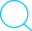# 计算机网络-传输层笔记

## TCP/IP

Posted by Kingtous on September 30, 2019

## 传输层

### TCP

• 优点
• 面向字节流
• 可靠性传输
• 细粒度
• 缺点
• 对于虚假的请求无法进行很好的防范
• DDOS攻击
• 开销大
• 鲁棒性

### TCP的传输效率

• 维持一个变量为MSS（最大报文段长度）
• 缓存大小大于MSS就发送
• 进程要求立即发送
• 计时器时限到了，就发送一波

• 若用户只发送1字节的东西，加上头部(20字节)尾部(20字节)构成的包将大大增加开销

• 尽量使用捎带的方法

Nagle算法：

• 如果app是逐个字节发到缓存中，则发送方先将第一个数据字节先发送出去，等待，收到确认后，再把缓存里所有数据发送出去

• 当到达的数据已经达到了发送窗口大小的一半或者已经达到了最大报文长度的一半时，就立即发送一个报文段

问题：[RFC 813,糊涂窗口综合征]接收方一次只接收一个字节（窗口设置很小，如为1）

• 让接收方等待一段时间，收到后先不立即发送报文，而是等待接收缓存里面已经有足够空间容纳一个最长的报文段或者接收缓存有一半的空间，才发送ACK确认

### RTT和RTO

RTT为最新测得的报文段的往返时间 $$New~RTT_s = (1- \alpha)\times (old~RTT_s)+\alpha \times (new~RTT)\\(0 \le \alpha < 1 ,~ \alpha_{recommand}=\frac{1}{8})$$ $RTT_D$表示为: $$new~RTT_D = (1-\beta)\times (old~RTT_D)+\beta\times |RTT_S - new~RTT|\\ \beta <1,~\beta_{recommand}=0.25$$ $RTT_D$表示为$RTT$的偏差的加权平均值。第一次时取为测量到的$RTT$样本值的一半.

$RTO(Reransform TimeOut):$ $$RTO = RTT_S + 4\times RTT_D$$ 延迟高问题：重传后收到了之前数据包的超时回复

• Karn算法：只要报文段重传了，这次就不用上述公式计算。
• 又带来问题：如果时延突然增加了很多，会导致重传，而RTT​和RTO不会更新
• 改进：重传一次，手动增加RTO(典型的做法为原RTO$\times 2$)